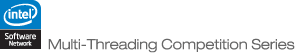JOINProblem Statement

### Problem Statement

You are attempting to decipher a message that is being received over an error prone communication line. All or part of the message may be received incorrectly. (See below for description of how errors are generated.)

Your class should implement one method, getTransmission, which takes two ints, N and C, the length of the message, and the number of distinct characters which may occur in the message (the first C uppercase letters). It will also take a double[], states, describing each possible state of the transmitter (explained below). Your getTransmission method may call the static method getMessage in class Message with two ints, beginPos and length, to request that a portion of the message be transmitted. You may call the getMessage method a maximum of N/4 times (except for the first 4 examples, where you are allowed 100 calls). (More calls than this will result in a 0 for the test case.) The getTransmission method should return a String containing the original message with as few errors as possible.

You will be allowed a total of 20 seconds execution time. There is a slight overhead during calls to getMessage, which is included in the runtime. However, you may have other threads running during the calls to the getMessage method.

Your score will be based upon count, the number of times the Message.getMessage method is called, transmit, the total number of characters that you request to be transmitted, and errors, the number of characters in your final return that are incorrect. The returned string must be the correct length or you will get a 0 for that test case. The raw score for each test case will be equal to 100 * N / (transmit + 10 * count + 1) / 2errors. In addition, a 2% penalty for each second of execution time will be applied to your score. In other words, if your program ran for 10 seconds, your final score would be your raw score minus 20%. Your total score will be the sum of your scores for each test case.

The manner in which errors are introduced into the transmission will be controlled by a finite state machine. At any given time, the communications line will be in one of several states each with an associated probability. If, when a character c is transmitted, the line is in a state with associated probability p, then the character c will be faithfully transmitted with probability p. With probability 1-p, however, one of the C characters (possibly c) will be transmitted at random (each with equal probability 1/C). After each character is transmitted, the communications line may switch into a different state or stay in the same state.

You will be given a double[] states indicating the associated probabilities for each of the communications line's states. The starting state for each transmission is equally likely to be any of the states. For each test, one additional value (not given), pChange, is the probability that, after a character is transmitted, the communications line changes to a different state (chosen randomly).

For example, imagine that states = { 0.1, 0.9 }. In this case, when the communications line is in state 0, characters are only transmitted correctly on occasion, while when the line is in state 1, they are almost always tranmitted correctly. If pChange were 0.01, then after each character was transmitted, the line would toggle to the other state with probability 0.01.

### Definition

 Class: MessageReceiver Method: getTransmission Parameters: int, int, double[] Returns: String Method signature: String getTransmission(int N, int C, double[] states) (be sure your method is public)

### Available Libraries

Class:Message
Method:getMessage
Parameters:int, int
Returns:String
Sample Call:val = Message.getMessage(beginPos, length);

### Notes

-To facilitate testing, the values of N and C were not chosen randomly for example cases 0 through 5.
-There are 100 non-example test cases.
-The memory limit is 1GB, while the thread limit is 32.

### Constraints

-The length of the message, N, will be between 1000 and 100000, inclusive. (chosen uniformly)
-The number of characters used in the message, C, will be between 2 and 26, inclusive. (chosen uniformly)
-For the state machine, the value of pCorrect for each state will be chosen uniformly between 0.1 and 1.0. The value of pChange (not given as input) will be chosen uniformly between 0.005 and 0.015.
-There are between 2 and 5 states, inclusive.

### Examples

0)

```N = 10, C = 4
states = {0.8422929341728342, 0.27001376084982964}

```
1)

```N = 20, C = 10
states = {0.8773517325544584, 0.2161027750118782, 0.280553917185094}

HEHFJCIJBFIJHBJAIGBG
```
2)

```N = 40, C = 8
states = {0.1365589320322015, 0.40725329056682824}

FDCCAACGGFGGGGBFDHGAAEGABHBBCBCFCFFABBBB
```
3)

```N = 100, C = 6
states = {0.8335353433161342, 0.6503830246420165}

BFECBDFEEAABEDBBACFFEDCCDEBFCABFCFCCDCCD
```
4)

```N = 1000, C = 26
states = {0.7757082451444491, 0.2599902835506756}

EUTRZVOUUOPPWYBXJXXBJMDEJNKJVRGDPDQMECBOXWOZCDWARFZUQWORIEMP
MIDEFSICXYDIWDZUWFXJCMYGPIMHQZOPGCAVPMWGMPXYNSMRZHFUFFGLATVH
PYNHTFAAKNNAGDHOFZRXDVPSTMBFBPQGIKXONGOXTVOIAMMHZHZOHYHGJQUN
DNBLKGGKJJJWQGTSENPWPGLTXOZUJFQRDHUZXSYRJPMWPCYVYISFVHJKEPMO
TTRIIIAPPZNNRYDCNXUEJLKAFKTGXANSJWDSXMBFGFCKUBNOHOOWSVECVZOX
JFGGTTUKLRKRRSWEMIQHOAAREOQLXDZEWILPXWDGTHNCSJLAVKQYZKXDGBKT
VPKSSEQBLDOTGTUDZZEZRYVWYSGNWDRDFYAVQSKIZJJWGJQFTOVNIZCDHKSE
NAITSLIUUNJWDAYPIYTWQHELJPGMWBUVXGTDOCHCRLPRBFQWYUGPYVHZEODS
YYVLGDAKKUSYYCXPRICICYCLGHGKJZCUZZQLCIISDDWCCUUGFVRUXHLKVVPS
NWNWBHRJTOGUFYYYOJFISMKELIRUSZFVOQRLIJSMYKYHDSHSIDIFHIPKTAIR
OFGRWSDTLMNMAJMCBJEZVXMAQJLREWHJKICCQYKCXBQRNCLNVNFIFTVOOKVM
WFMUFHRROIXYMUHMNXVRFOMGBARXKNSUWPVBQMYAZZMAEMAODCZRLBTYHYGW
GJWIXGCBMTOILWGCSTSKLWNKUXGMJGKKJSXYRJSYKGODOBFDXRPRGCHKGNAA
KUWBTWVGPUZJXUDNIJZPERSDVWLMCRAYTYWKQUOTEMKZQXNFYEKEBFONMIBT
ZYOEXCCNIIHJUHDRJHJGPTTZBUDVKUAFLSPUCFWVCTYOLZAIOHXEAHMEWXAO
NEQATGLKHCDCBTFMOHEGCECEVTWDOIIXOPHWMZHU
```
5)

```N = 10000, C = 26
states = {0.9748383017983552, 0.19013704142162913}

```
6)

```N = 62470, C = 18
states = {0.15397192389252276, 0.289645795623697, 0.8190145293608756, 0.8794922843679077}

```
7)

```N = 6555, C = 9
states = {0.5313863217801227, 0.4363762842450074, 0.6280224192300305}

```
8)

```N = 65894, C = 6
states = {0.8053024200739403, 0.5683403306949268, 0.540334947695794, 0.3165917779909993}

```
```N = 14849, C = 7